Department of Management Science & Engineering

Number 10/Spring 2002

Forum

CONTENTS

Forum

Powerful Project Pricing

We are pleased by the substantial interest in these Newsletters and in the associated website http://www.investmentscience.com. Many of you now receive an automatic notification when a new issue of the Newsletter is posted.

The technical article in this issue presents a new, powerful pricing method that is intuitive, easy to implement, and consistent with the most advanced theoretical methods. You can begin to use this technique immediately.

Our research at Stanford University and our applications to practical problems has been expanding into issues well beyond those that were considered when the first issue of this Newsletter was published several years ago. In addition to evaluation of uncertain (risky) project cash flow streams and real options, we have applied investment science to product pricing, R&D portfolio formulation, product portfolio analysis, and various business strategy issues. Many of you readers have been participants in these applications, and we have enjoyed working with you.

We have found that giving the short course in a company can be an effective way to get a team understanding of the concepts of investment science. This facilitates the acceptance of the methods as the best way to view project design and other decisions with major financial impact. If you are interested in having the short course in your company, contact me.

Powerful Project Pricing

Pricing of a project fundamentally rests on evaluation of the cash flows that the project will generate. If these cash flows are known with certainty at the time of project conception the value of the project can be determined by a discounted cash flow analysis. But, the cash flow stream of most projects cannot be determined with certainty ahead of time. Future cash flows are uncertain and this is why a different pricing theory is needed.

Modifications of discounted cash flow analysis are available, including the popular Capital Asset Pricing Model (CAPM), which is based on the relation of the cash flows of the project to an overall market index. The relation is summarized by the parameter "beta" that is familiar to those in the finance field. Although the CAPM is a benchmark for many theoretical discussions, its use as a method for assigning value has been somewhat limited because it is not easy to determine the value of beta for a project.

A more popular (and simpler) method is the method known as comparables, which is intuitive, relatively simple, and fairly reliable. In this method an asset's value is estimated by comparing it with similar assets with known prices. For example, when attempting to determine the value of a house, one generally looks at recent sales of similar houses in the same neighborhood. Likewise, when evaluating the value of a potential company acquisition, a firm finds comparable companies that have recently been purchased. Indeed, the concept of using comparables is one of the oldest and most accepted methods of price determination.

There appears to be a distinct difference between the highly analytical method of the traditional CAPM and the looser, more intuitive, method of comparables. However, our recent work in investment science has forged a simple link between the two, making the CAPM value easier and more intuitive, and making the method of comparables more robust. This new method, called the correlation pricing formula, is described below.

Correlation Pricing Formula

Suppose that an asset has its payoff in exactly one year. The payoff is denoted y but it is uncertain. It can be characterized by its expected (or mean) value E(y) and its standard deviation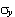. A first approximation to the value at the current time of a payoff y one year later is v = E(y)/R where R is the total return on a risk free asset (such as a T-bill). This merely discounts the mean value by the risk free rate.

To account for the fact that y is uncertain and therefore risky, some people recommend discounting by a higher rate, and often they suggest that the greater the uncertainty (the), the greater the discount rate. However, the CAPM formula does not increase the discount rate, it decreases the numerator. The exact formula is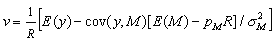In this formula M is the payoff of a special "market" portfolio. Normally, it is taken to be the payoff of a market index such as the S&P 500. The term cov(y,M) is the covariance of y with M,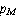is the current price of the market portfolio, and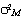is the variance of M.
*
The important point of the CAPM is that the economic value of y does not depend on the standard deviationbut rather on "beta" defined as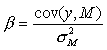In particular, we note that if y is independent of the market portfolio M, then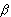= 0 and we find the simple formula v = E(y)/R.

In practice, it is difficult to findfor project investments. For example, it would be difficult to evaluate a house by determining its beta on the basis of the next year's value and some general housing index. Likewise, it would difficult to determine the value of a start up company by finding its beta relative to the S&P 500.

The correlation pricing formula (CPF) has exactly the same form as the CAPM but with one critical difference. The formula is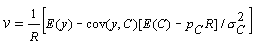where now C is the payoff of an existing priced (or marketed) asset most correlated with y. For example, in pricing a house, C might be the payoff of the house next door which sold last week. If we thought the two houses were virtually identical, then y =C and the formula would reduce to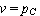. If we thought that our house was about half-way in between two other houses in terms of its value characteristics, then the price would be the average of the prices of these two other houses.

Sometimes a more complex analysis is required. For example, if we value a project by its cash flow, we need to relate the cash flow of the project to that of the most correlated priced asset (such as a company that has similar projects) or to an appropriate combination of such assets. All of this is likely to be easier and more precise than attempting to relate the new house or project to a general market index.

The surprising and important result is that this correlation pricing formula gives precisely the same result as would the CAPM formula if both were computed exactly. That is, if the exact beta of our project were known and entered into the CAPM formula, and if the most correlated asset were known and related to the project to find the CPF price, then both would agree. Hence, the correlation pricing formula is not arbitrary. It is a solid link between the theoretical CAPM and the intuitive and easily implemented method of comparables. The method can be easily extended to the case where cash flows occur at several periods.

Example. Your company has value equal to

Value = Debt + Equity.

Suppose that debt represents a fraction a of the value and equity represents a fraction 1 - a. Then the value V can be written asto reflect the division into debt and equity. One year from now the value will bewhere R is the total risk free return (which increases the debt) and S is the (random) return on the equity which is the return on the stock.

Your company keeps a fraction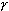of its value in cash and invests a fraction 1 -in projects. Hence the value next year is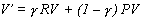where P is the (random) return on projects.

By equating the two expressions for V' we find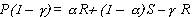and hence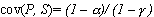.

Now suppose that you have a project that is much like every other project in the firm; that is, its random payoff Q is similar in character to other projects. In that case, you can take the stock of your company to be a most correlated marketed asset. The payoff of your project is of the form Q = qP and thus the value of your project is according to the CPF equal to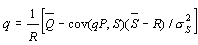Solving for q we have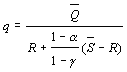Hence you may find the value of your (typical) project by discounting its expected value by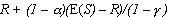As a special case, suppose that a == 0, which means that the company has no debt and all value is invested in projects. In that case the discount factor in the denominator is E(S), which means that you discount by the rate of growth of the stock. This is, of course, exactly the principle of how market risk is discounted, for in this special case the project is exactly copying the market in terms of risk.

As another special case, suppose that= 0 but a is not zero. This means there is debt but all value is invested in projects. In this case, the discount factor in the denominator is, which is termed the weighted average cost of capital WACC, reflecting the value equation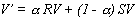A most interesting case is when> a which means that your company has more cash than debt. Lots of cash and no debt is often considered the sign of a healthy company, and maybe it is. But in the single period framework of this analysis, it means that projects must be discounted more heavily than the WACC, and even more heavily than the stock if there is no debt. The relatively small proportion of projects must carry a heavy load, exhibiting high returns, to enable the company's stock to grow appropriately when burdened by cash that earns a low return.

Of course, if your project is not of the same character as other projects you can modify this analysis to find the appropriate discount factor.

Reference: Luenberger, David G. "A Correlation Pricing Formula", Journal of Economic Dynamics and Control, 26, (2002), 1113-1126. v